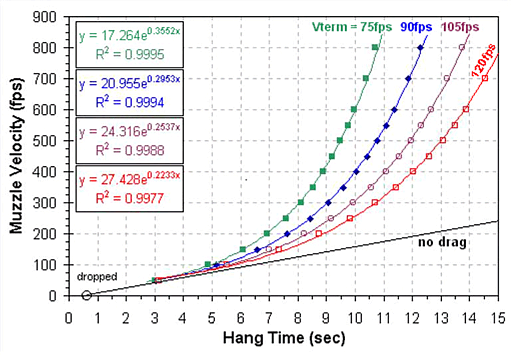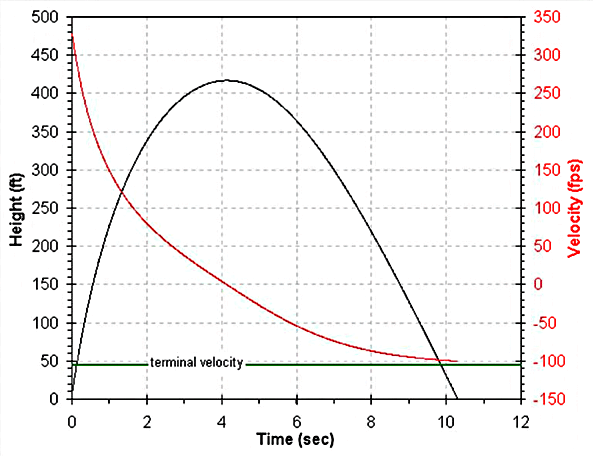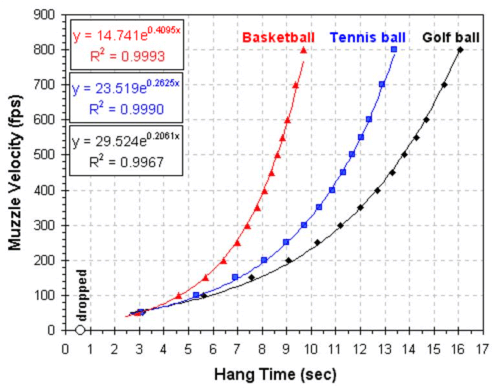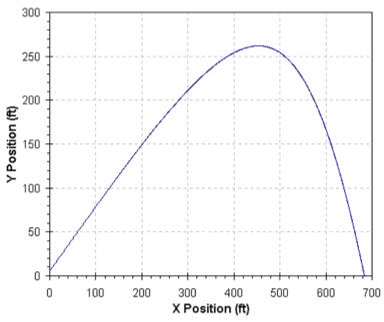# Estimating Muzzle Velocity from Hang Time

Updated June 5, 2007

One method for estimating the muzzle velocity of a spud gun is to measure the hang time for a spud fired straight up. The "hang time" is the time from when the spud leaves the barrel until it hits the ground. If there is no air friction, then the speed at which the spud hits the ground is the same as the muzzle velocity. If air friction is neglected, the formula is based on the equation of motion in a uniform gravitational field. Starting from

Fgravity = mg                               (1)

Muzzle velocity = 1/2 gt              (2)

Where g is the acceleration due to gravity (32 fpss) and t is the hang time.  Unfortunately, for a typical spud gun, air friction is significant and makes the simple calculation fail miserably.

## Including Air Resistance

Calculating trajectories that include air friction is more complicated. The most common treatment of air friction for projectiles in this speed domain is non-viscous friction;

Ffriction = kv2                               (3)

Where v is the velocity and k is a frictional factor. If we combine the Ffriction equation with the Fgravity equation we get an equation for the total force acting on our spud;

Ftotal = Fgravity + Ffriction = mg + kv2 = matotal           (4)

atotal = g + (kv2/m)                                                   (5)

Where Ftotal is the net force on the spud and atotal is the net acceleration. Unfortunately, this is a second degree differential equation and I am to dense to solve the equations of motion. Instead, I separated the X and Y components of motion and treated the equations at discrete time steps.

## k and the Terminal Velocity of a Spud

The first challenge is coming up with a suitable frictional factor (k) for a spud. One approach to estimating k is to calculate it from the terminal velocity of a spud. At terminal velocity,

Fgravity = Ffriction                    (6)

atotal = 0                               (7)

mg = kvterminal2                     (8)
k = mg/vterminal2                    (9)

If we know the mass, acceleration due to gravity and terminal velocity (vterminal), we can calculate k. So, what is the terminal velocity of a spud? A search of the web didn't turn up the terminal velocity (or k) of a spud, but I did find the terminal velocities of baseballs and golf balls. Baseballs and golf balls both have terminal velocities of ~105fps (32m/s) or ~135fps (41m/s) depending on the data source.

## More than you really wanted to know about Drag and the Frictional Factor

Mathematically, terminal velocity (Vterminal) can be calculated from;

vterminal = Sqrt(2mg/ρACd)            (10)

Where

m is the mass of the falling object,
g is gravitational acceleration at the Earth's surface (32 fpss, 9.8 m/s2),
Cd is the drag coefficient,
ρ is the density of the fluid the object is falling through (~1.28kg/m3 for air),
A is the object's cross-sectional area.

The equation above would allow us to calculate the terminal velocity of our spud if we know the mass, cross sectional area and drag coefficient (Cd). You'll notice that this equation contains an m/A (mass over cross-sectional area) term. This term is the sectional density of the projectile. The sectional density of a projectile describes how much of an affect drag will have on its velocity. Projectiles with large sectional densities are affected less by air drag. For a spherical projectile, the sectional density does not change as (if) it tumbles. For a cylindrical or bullet shaped projectile like a spud, the sectional density changes as the projectile tumbles. The table below gives values for the diameter, mass and sectional density of baseballs, golf balls and for a 2" diameter by 3" long "bullet shaped" spud.

 Ammo Diameter (inch) Mass (g) Sectional Density (g/in2) (Note 1) Baseball 2.9 144 22 Golf ball 1.68 46 21 Spud (end-on flight) (Note 2) 2 ~100 32 Avg. = 28 Spud (sideways flight) (Note 2) 3 ~100 25

Note 1: Calculated as mass/area which is somewhat different than the more common mass/radius2 or mass/diameter2.

Note 2: For a half spud calculated as a 2"diameter by 1" long cylinder plus a 2" diameter by 1.5" long paraboloid. Volume = 5.5in3 (90cm3), frontal area = 3.14in2, side area = 4.0in2, desntiy of a spud is ~1.1g/cm3 (18g/in3).

As you can see from the table, the sectional densities of baseballs and golf balls are nearly identical. Since they both have the same shape (spheres) they would be expected to have about the same terminal velocities. Literature values for the vterminal of baseballs and golf balls are 138fps (42m/s) and 132fps (40m/s), respectively.

The sectional density of a cylindrical hunk of spud is more difficult to get a handle on. A spud fired from a smooth barrel will tumble in flight. As a result, the sectional density will change depending on whether it is flying "end-on" (like it would if it didn't tumble) or sideways. For the lack of a better way to treat the sectional density of a cylindrical shell that tumbles I just assume that the average of the two sectional densities is a reasonable value. The average is 28 g/in2,  roughly a third more than the sectional density of baseballs and golf balls.

So, the equation for vterminal can be rewritten using the sectional density (Ds) instead of m and A;

vterminal = Sqrt(2Dsg/ρCd)                   (11)

We now need a value for the coefficient of drag, Cd. The Cd of some basic shapes are listed in the table below (values from here.) Note that Cd is a dimension less number, that is, it has no units.

 Cd Shape 2.1 a smooth brick 1.2 person (upright position) 0.9 a typical bicycle plus cyclist 0.4 rough sphere (Re = 106) 0.1 smooth sphere (Re = 106)

Baseballs and golf balls probably have Cd values in the range of the rough and smooth spheres, 0.1 to 0.4. But what about a cylindrical spud? Perhaps something approaching the value for a "smooth brick"? It seems reasonable that the Cd of the spud is in the range of perhaps three to 10 times greater than for a baseball or golf ball. As the Cd of the spud increases the terminal velocity decreases.

So comparing a spud to a baseball or golf ball, the spud has a ~33% higher sectional density, which increases the terminal velocity, but a three to ten times greater coefficient of drag, which decreases terminal velocity.

Comparing equations 9 and 10 lets us define k.

k = mg/vterminal2                       (9)

Vterminal = Sqrt(2mg/ρACd)        (10)

So,

k = ρACd /2                               (12)

In the absence of better estimates for k or Cd, I figure a value in the range of  75 to 120 fps is a reasonable estimate for the terminal velocity of your average spud. Note that the terminal velocity is quite a bit less than your typical spud gun muzzle velocities of 200 to 600 fps. That is why the simple equation for calculating the muzzle velocity from hang time fails so badly. The spud starts at the muzzle velocity (typically quite a bit greater than the terminal velocity), but on the way back down its speed can never exceed its terminal velocity.

Plugging vterminal = 90fps into the equation for k (9), and assuming a typical spud from a 2" barrel has a mass of 0.265 pound (120g), gives an estimate for k of 0.0010469 (lbs mass)/ft. k values for for 75, 90, 105 and 120fps terminal velocities are shown in the table below.

 vterminal (fps) k (lbs mass/ft) k (kg/m) 75 0.0015076 0.00224 90 0.0010469 0.00156 105 0.0007692 0.00114 120 0.0005889 0.00088

## Estimating Muzzle Velocity from Hang Time Including Air Friction

I have calculated the hang time for various muzzle velocities using equations derived from the Ftotal equation. The various values used are given in the table below.

 Variable Value Units Comments m 0.265 pounds (as mass) spud mass (0.265 lb = 120g), approximate mass of a half spud in a 2" diameter barrel k 0.00151 pounds (as mass)/ft frictional constant estimated assuming 75fps terminal velocity and m=0.265) 0.00104 frictional constant estimated assuming 90fps terminal velocity and m=0.265) 0.000769 frictional constant estimated assuming 105fps terminal velocity and m=0.265) 0.000589 frictional constant estimated assuming 120fps terminal velocity and m=0.265) theta 90 degrees barrel inclination (90º = straight up) g 32 ft/sec2 acceleration due to gravity Yzero 6 ft height of muzzle above ground dT 0.003 sec time slice for calculations

The graph below shows the muzzle velocity plotted against the hang time using three values of k corresponding to terminal velocities of 75, 90, 105 and 120 fps. These curves were calculated for a 120 g (0.265 lb) pound spud launched vertically from a height of 6 feet. The black curve is calculated ignoring air friction. As you can see, at typical spud velocities, there is a big difference between the muzzle velocity calculated from hang times with and without air resistance. For example, for a hang time of 10 seconds and assuming a terminal velocity of 90 fps, the simple calculation indicates a muzzle velocity of 160fps but including air resistance (drag) gives a muzzle velocity of 400fps.## Calculations

These calculations were done as an Excel spread sheet. the equations were:

 Y Coordinates X Coordinates Initial: (at time = 0) Y0 = muzzle height X0 = 0 vy,0 = (muzzle velocity)*SIN(theta*Pi/180) Vx,0 = (muzzle velocity)*COS(theta*Pi/180) a y,0 = -g - k*vy,02/m a x,0 = -k*X vx,02/m Incremental: (at time = i) Yi = Yi-1 + dT*vy,i-1 X i = X i-1 + dT*vx,i-1 vy,i = vy,i-1 + dT*ay,i-1 vx,i = vx,i-1 + dT*a x,i-1 ay,i = -g - SIGN(vy,i)*k*vy,i2/m a x,i = -k*vx,i2/m

Where X and Y are the horizontal and vertical positions, v is velocity and a is acceleration. The first subscript on the v and a terms give the axis (X or Y). The other subscript is the time slice. dT is the length of the time slice.

A graph of height (black) and velocity (red) versus time is shown below for a spud launched straight up. The green line marks the terminal velocity (-105fps). This graph is for an 0.265 pound (120g) spud, a muzzle velocity of 330fps and a k value based on a terminal velocity of 105fps.## Other Projectiles

There are many other projectiles that a spudder might decide to launch. The table below lists various parameters of several that might be of interest.

 Object Terminal  Velocity, m/s (fps) Diameter, cm (inch) Mass, g (oz) Cross Sectional  Area, cm2 Sectional  Density, g/cm2 Cd , (unitless) (note 1) k , g/m (note 2) k , (lb mass /ft) (note 2) 16-lb Shot 145 (476) 12 (4.72) 7260 (256) 113 6.42 0.464 3.38 2.26x10-3 Baseball 42 (138) 7.4 (2.90) 145 (5.11) 43 3.37 0.290 0.806 5.37x10-4 Golf ball 40 (132) 4.27 (1.68) 45 (1.59) 14.3 3.16 0.299 0.276 1.82x10-4 Softball 36 (117) 9.7 (3.82) 181 (6.38) 74 2.45 0.287 1.37 9.33x10-4 Handball (note 4) 34 (110) 4.78 (1.88) 64 (2.26) 18 3.54 0.467 0.543 3.73x10-4 0.177" Copper / Steel BB ? 0.45 (0.177) 0.33 (0.012) 0.159 2.08 ? ? ? Tennis Ball 31 (102) 6.5 (2.56) 59 (2.08) 33 1.78 0.283 0.602 4.00x10-4 Soccer Ball 25 (81) 22.2 (8.75) 426 (15.0) 387 1.1 0.268 6.68 4.58x10-3 Basketball 20 (66) 24.1 (9.5) 600 (21.2) 456 1.32 0.500 14.7 9.72x10-3 Volleyball 16 (51) 21.4 (8.43) 254 (8.96) 360 0.71 0.419 9.72 6.89x10-3 Ping-Pong Ball 9 (30) 3.7 (1.47) 2.7 (0.0952) 10.8 0.25 0.460 0.327 2.12x10-4 Raindrop (1.5mm r) 7 (23) 0.3 (0.12) 0.014 (0.00049) 0.071 0.2 0.611 0.0028 1.87x10-6

Table Notes:

1. Cd calculated using Cd=2mg/(vterminal2) from equation 10 with g=9.8m/s and ρ=1290 g/m3.
2. k calculated using equation 9 with g=32ft/s2.
3. See the links section below for references.
4. There appears to be some problem with the data for Handballs. The terminal velocity may be closer to 44m/s
and the Cd ~ 0.3.

The graph below shows the muzzle velocity as a function of hang time for basketballs, tennis balls and golf balls.The graph above was calculated using the values in the table above and these parameters;

 theta 90 degrees barrel inclination (90 = straight up) g 32 ft/sec^2 acceleration due to gravity Yzero 6 ft muzzle height Tstep 0.003 sec time slice

## Other Things That Can Be Calculated

Once the equations of motion and a value of k are available, there are several other things that can be calculated.

### Range

Using the equations for the X-Coordinates, the range of the spud for a given muzzle velocity, spud mass and launch angle can be calculated.

### Optimal Launch Angle

Using a fixed muzzle velocity, the launch angle that gives the maximum horizontal distance (range) can be calculated. For a projectile in a vacuum, the maximum range is achieved with a 45° launch angle.

For an 0.265 pound spud at 330fps muzzle velocity with k based on a 105fps terminal velocity, the maximum range is 684ft at a 36° launch angle.## Doing a Better Job

To do a better job on these calculations I really need a set of data consisting of muzzle velocities (measured with a chronometer), spud masses and hang times. From this data a better estimate of k can be obtained and the equations can be checked against reality.

## Questions & Issues

• Affect of different barrel diameters (2", 1.5" ...)? Does k change much or is the change in mass sufficient for a reasonably accurate result?
• Does a rifled barrel have much of an affect on k? (If the spud doesn't tumble then the Cd and Ds values will be different.)
• How variable is the mass of your typical spud projectile?

## Things Neglected

• Differences in air density, temperature, humidity ...
• Affect of approaching the speed of sound
• Wind